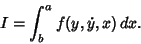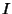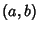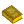## Calculus of Variations

A branch of mathematics which is a sort of generalization of Calculus. Calculus of variations seeks to find the path, curve, surface, etc., for which a given Function has a Stationary Value (which, in physical problems, is usually a Minimum or Maximum). Mathematically, this involves finding Stationary Values of integrals of the form(1)has an extremum only if the Euler-Lagrange Differential Equation is satisfied, i.e., if(2)

The Fundamental Lemma of Calculus of Variations states that, if(3)

for allwith Continuous second Partial Derivatives, then(4)

on.

See also Beltrami Identity, Bolza Problem, Brachistochrone Problem, Catenary, Envelope Theorem, Euler-Lagrange Differential Equation, Isoperimetric Problem, Isovolume Problem, Lindelof's Theorem, Plateau's Problem, Point-Point Distance--2-D, Point-Point Distance--3-D, Roulette, Skew Quadrilateral, Sphere with Tunnel, Unduloid, Weierstraß-Erdman Corner Condition

ReferencesCalculus of Variations

Arfken, G. Calculus of Variations.'' Ch. 17 in Mathematical Methods for Physicists, 3rd ed. Orlando, FL: Academic Press, pp. 925-962, 1985.

Bliss, G. A. Calculus of Variations. Chicago, IL: Open Court, 1925.

Forsyth, A. R. Calculus of Variations. New York: Dover, 1960.

Fox, C. An Introduction to the Calculus of Variations. New York: Dover, 1988.

Isenberg, C. The Science of Soap Films and Soap Bubbles. New York: Dover, 1992.

Menger, K. What is the Calculus of Variations and What are Its Applications?'' In The World of Mathematics (Ed. K. Newman). Redmond, WA: Microsoft Press, pp. 886-890, 1988.

Sagan, H. Introduction to the Calculus of Variations. New York: Dover, 1992.

Todhunter, I. History of the Calculus of Variations During the Nineteenth Century. New York: Chelsea, 1962.

Weinstock, R. Calculus of Variations, with Applications to Physics and Engineering. New York: Dover, 1974.